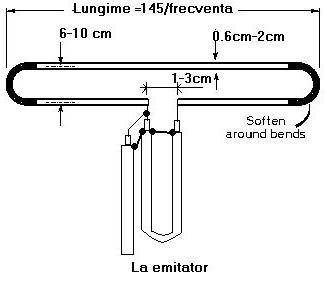EXPERIMENT NO.2

Analysis of Folded Antenna

 Time ( min) Content Learning Aid / Methodology Faculty Approach Typical Student Activity Skill / Competency Developed 10 Relevance and significance of experiment Chalk & Talk , Presentation Introduces, Facilitates, Monitors Listens, Participates, Discusses Knowledge, Communication, intrapersonal 20 Explanation of experiment Chalk & Talk , Presentation Introduces, Facilitates, Explains Listens Knowledge, Communication, intrapersonal, Application 60 Reading Role play/ demonstration Explains, Monitors Participates, Discusses Debate, comprehension, Hands on experiment 20 Calculations N/A Explains, Monitors Participates, Discusses Knowledge, Communication, Intrapersonal, Application 10 Results and conclusions Keywords Lists, Facilitates Listens, Participates, Discusses Knowledge, Communication, intrapersonal, Comprehension

Practical Session Plan

Title: Analysis of Folded Antenna

Objectives: Mount and set up the connections of folded antenna to analyze the performance parameters

Aim: 1) To sketch the radiation pattern of folded dipole antenna.

2) To Compute gain, beam width, return loss & impedance of folded Dipole antenna

Apparatus: Antenna, transmitter, receiver-stepper motor controller, dipole antenna, folded dipole antenna stepper pod, connecting cables, direction coupler, slotted line, 20 db attenuator etc.

Theory: Another common place one can see dipole is as antennas for the FM band - these are folded dipoles. The tips of the antenna are folded back until they almost meet at the feed point, such that the antenna comprises one entire wavelength. This arrangement has a greater bandwidth than a standard half-wave dipole. If the conductor has a constant radius and cross-section, at resonance the input impedance is four times that of a half-wave dipole.Procedure

A) To sketch the radiation pattern of folded dipole antenna in azimuth plane on polar plots.

1) Connect the dipole antenna to the tripod & set the transmitter frequency to 600 MHz

2) The length of antenna is c/2f=(3*10^8)\(2*600*10^6)=25cm end to end.

3) Connect another folded dipole antenna to stepper pod & set the receiver to 600 MHz +.Connect 20dB

attenuator at receiver side to avoid saturation & set the length of receiver dipole antenna same as

25cm at both ends.

4) Rotate the folded dipole antenna of receiver end around its axis in steps of using stepper motor

controller .

rotation of antenna to the corresponding memory location .

6) Connect RS232 from receiver to computer & transfer the reading by enabling serial mode at receiver .

Hence radiation pattern is plotted on polar plot from which gain & beam width is obtained.

7) Beam width is obtained on log plot at 3dB level.

B) Calculation of return loss using direction coupler:

1) Connect the direction coupler to the transmitter tripod to RF out connector .

2) Connect the antenna to the direction coupler to RF in connector.

3) Connect the receiver to the direction coupler at RF sample connector and insert attenuator.

4) Bring the transmitter & receiver to 600MHz and take the reading this will give forward power .

5) Now interchange the connection of transmitter tripod & antenna is connected at RF sample.

6) Now the transmitter tripod is at RFin and antenna is connected at RFout .

7) Note down the reading at receiving at same frequency (600MHz) this will give reverse power.

8) The return loss is hence obtained by Return loss=forward power-reverse.

C) Calculation of Imprdance:-

1. Connect generator at 0 cm pointof slotted line . Set the frequency of source as 600 MHz. Hence free

space wavelength lambda =20cm.

2. Connect short load at 20cm end of slotted line.

3. Move the probe from 0cm to 20cm that is generator to load end of line.

4. Observing Rx reading that goes Max to Min again Min to Max. Hence with short connected 1st minima

is at & 2nd minima is at .

5 . Now connect unknown load ( antenna) at 20cm end & measuring shift in minima. Observing

Whether minima has shifted towards load end or generator end.

6 . Thus with antenna connected at load end, 1st minima occurs at cm & 2nd occurs at cm.

7. Hence w.r.t short on slotted line the minima has shifted by cm towards load end when antenna is

Replaced with short.

8. Hence shift in minima /lambda =

9. For calculating VSWR, using directional coupler measuring Return loss of antenna.

10. Now using formula for calculating Reflection coefficient which is

R.L. = -20log

11. After calculating , calculating VSWR using formula

VSWR = 1+ / 1-

This gives VSWR which is

12 . Draw a circle on Smith chart with radius taking center of smith chart. This circle is called constant

VSWR circle.

13 With ratio of shift in minima /lambda = , rotating anticlockwise from left & locate on outer circle.

14 . Draw a line connecting to center of circle such that it crosses VSWR circle. This point is read as

as real part & as imaginary part.

15 . Hence normalized impedance is

16. Actual impedance is ( ) 50 = ohms.

Observations:

Sample Calculations:

Results:

1) Max.Gain=

2) Beam width=

3) Forward power=

4) Reverse power=

5) Return loss=-

6) Impedance=